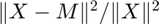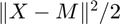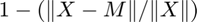# All-at-once optimization for CP tensor decomposition

We explain how to use cp_opt function which implements the CP-OPT method that fits the CP model using direct or all-at-once optimization. This is in contrast to the cp_als function which implements the CP-ALS method that fits the CP model using alternating optimization. The CP-OPT method is described in the following reference:

• E. Acar, D. M. Dunlavy and T. G. Kolda, A Scalable Optimization Approach for Fitting Canonical Tensor Decompositions, J. Chemometrics, 25(2):67-86, 2011, http://doi.org/10.1002/cem.1335

This method works with any tensor that support the functions size, norm, and mttkrp. This includes not only tensor and sptensor, but also ktensor, ttensor, and sumtensor.

## Major overhaul in Tensor Toolbox Version 3.3, August 2022

The code was completely overhauled in the Version 3.3 release of the Tensor Toolbox. The old code is available in cp_opt_legacy and documented here. The major differences are as follows:

1. The function being optimized is nowwhere X is the data tensor and M is the model. Previously, the function being optimized was. The new formulation is only different by a constant factor, but its advantage is that the convergence tests (e.g., norm of gradient) are less sensitive to the scale of the X.
2. We now support the MATLAB Optimization Toolbox methods, fminunc and fmincon, the later of which has support for bound contraints.
3. The input and output arguments are different. We've retained the legacy version for those that cannot easily change their workflow.

## Optimization methods

The cp_opt methods uses optimization methods from other packages; see Optimization Methods for Tensor Toolbox. As of Version 3.3., we distribute the default method ('lbfgsb') along with Tensor Toolbox.

Options for 'method':

• 'lbfgsb' (default) - Uses L-BFGS-B by Stephen Becker, which implements the bound-constrained, limited-memory BFGS method. This code is distributed along with the Tensor Toolbox and will be activiated on first use. Supports bound contraints.
• 'fminunc' or 'fmincon' - Routines provided by the MATLAB Optimization Toolbox. The latter supports bound constraints. (It also supports linear and nonlinear constraints, but we have not included an interface to those.)
• 'lbfgs' - Uses POBLANO Version 1.2 by Evrim Acar, Daniel Dunlavy, and Tamara Kolda, which implemented the limited-memory BFGS method. Does not support bound constraints, but it is pure MATLAB and may work if 'lbfgsb' does not.

## Optimization parameters

The full list of optimization parameters for each method are provide in Optimization Methods for Tensor Toolbox. We list a few of the most relevant ones here.

• 'gtol' - The stopping condition for the norm of the gradient (or equivalent constrainted condition). Defaults to 1e-5.
• 'lower' - Lower bounds, which can be a scalar (e.g., 0) or a vector. Defaults to -Inf (no lower bound).
• 'printitn' - Frequency of printing. Normally, this specifies how often to print in terms of number of iteration. However, the MATLAB Optimization Toolbox method limit the options are 0 for none, 1 for every iteration, or > 1 for just a final summary. These methods do not allow any more granularity than that.

## Simple example problem #1

Create an example 50 x 40 x 30 tensor with rank 5 and add 10% noise.

```rng(1); % Reproducibility
R = 5;
problem = create_problem('Size', [50 40 30], 'Num_Factors', R, 'Noise', 0.10);
X = problem.Data;
M_true = problem.Soln;

% Create initial guess using 'nvecs'
M_init = create_guess('Data', X, 'Num_Factors', R, 'Factor_Generator', 'nvecs');
```

## Calling cp_opt method and evaluating its outputs

Here is an example call to the cp_opt method. By default, each iteration prints the least squares fit function value (being minimized) and the norm of the gradient.

```[M,M0,info] = cp_opt(X, R, 'init', M_init, 'printitn', 25);
```
```CP-OPT CP Direct Optimzation
L-BFGS-B Optimization (https://github.com/stephenbeckr/L-BFGS-B-C)
Size: 50 x 40 x 30, Rank: 5
Lower bound: -Inf, Upper bound: Inf
Parameters: m=5, ftol=1e-10, gtol=1e-05, maxiters = 1000, subiters = 10

Begin Main Loop
Iter    25, f(x) = 5.529663e-02, ||grad||_infty = 2.18e-04
Iter    50, f(x) = 9.818485e-03, ||grad||_infty = 1.78e-04
Iter    55, f(x) = 9.809476e-03, ||grad||_infty = 3.71e-06
End Main Loop

Final f: 9.8095e-03
Setup time: 0.016 seconds
Optimization time: 0.33 seconds
Iterations: 55
Total iterations: 161
```

It's important to check the output of the optimization method. In particular, it's worthwhile to check the exit message. The message CONVERGENCE: REL_REDUCTION_OF_F_<=_FACTR*EPSMCH means that it has converged because the function value stopped improving. The message CONVERGENCE: NORM_OF_PROJECTED_GRADIENT_<=_PGTOL means that the gradient is sufficiently small.

```exitmsg = info.optout.exit_condition
```
```exitmsg =

```

The objective function here is different than for CP-ALS. That uses the fit, which is. In other words, it is the percentage of the data that is explained by the model. Because we have 10% noise, we do not expect the fit to be about 90%.

```fit = 1 - sqrt(info.f)
```
```fit =

0.9010

```

We can "score" the similarity of the model computed by CP and compare that with the truth. The score function on ktensor's gives a score in [0,1] with 1 indicating a perfect match. Because we have noise, we do not expect the fit to be perfect. See doc score for more details.

```scr = score(M,M_true)
```
```scr =

0.9986

```

## Specifying different optimization method

```[M,M0,info] = cp_opt(X, R, 'init', M_init, 'printitn', 25, 'method', 'fminunc');
```
```CP-OPT CP Direct Optimzation
Unconstrained Optimization (via Optimization Toolbox)
Size: 50 x 40 x 30, Rank: 5
Parameters: gtol=1e-05, maxiters = 1000, maxsubiters=10000

Begin Main Loop

Local minimum found.

Optimization completed because the size of the gradient is less than
the value of the optimality tolerance.

End Main Loop

Final f: 9.8097e-03
Setup time: 1.7 seconds
Optimization time: 2.5 seconds
Iterations: 107
Total iterations: 1004
Exit condition: Gradient norm smaller than tolerance
```

Check exit condition

```exitmsg = info.optout.exit_condition
% Fit
fit = 1 - sqrt(info.f)
```
```exitmsg =

fit =

0.9010

```

## Calling cp_opt method with higher rank

Re-using the same example as before, consider the case where we don't know R in advance. We might guess too high. Here we show a case where we guess R+1 factors rather than R.

```% Generate initial guess of the correct size
rng(2);
M_plus_init = create_guess('Data', X, 'Num_Factors', R+1, ...
'Factor_Generator', 'nvecs');
```
```% Run the algorithm
[M_plus,~,output] = cp_opt(X, R+1, 'init', M_plus_init,'printitn',25);
exitmsg = info.optout.exit_condition
fit = 1 - sqrt(info.f)
```
```CP-OPT CP Direct Optimzation
L-BFGS-B Optimization (https://github.com/stephenbeckr/L-BFGS-B-C)
Size: 50 x 40 x 30, Rank: 6
Lower bound: -Inf, Upper bound: Inf
Parameters: m=5, ftol=1e-10, gtol=1e-05, maxiters = 1000, subiters = 10

Begin Main Loop
Iter    25, f(x) = 5.530646e-02, ||grad||_infty = 2.50e-04
Iter    50, f(x) = 9.803871e-03, ||grad||_infty = 8.52e-05
Iter    56, f(x) = 9.785758e-03, ||grad||_infty = 5.15e-06
End Main Loop

Final f: 9.7858e-03
Setup time: 0.0026 seconds
Optimization time: 0.16 seconds
Iterations: 56
Total iterations: 163

exitmsg =

fit =

0.9010

```
```% Check the answer (1 is perfect)
scr = score(M_plus, M_true)
```
```scr =

0.9986

```

## Simple example problem #2 (nonnegative)

We can employ lower bounds to get a nonnegative factorization. First, we create an example 50 x 40 x 30 tensor with rank 5 and add 10% noise. We select nonnegative factor matrices and lambdas. The create_problem doesn't really know how to add noise without going negative, so we hack it to make the observed tensor be nonzero.

```R = 5;
rng(3);
problem2 = create_problem('Size', [50 40 30], 'Num_Factors', R, 'Noise', 0.10,...
'Factor_Generator', 'rand', 'Lambda_Generator', 'rand');
X = problem2.Data .* (problem2.Data > 0); % Force it to be nonnegative
M_true = problem2.Soln;
```

## Call the cp_opt method with lower bound of zero

Here we specify a lower bound of zero with the last two arguments.

```[M,M0,info] = cp_opt(X, R, 'init', 'rand','lower',0,'printitn',25);

% Check the output
exitmsg = info.optout.exit_condition

% Check the fit
fit = 1 - sqrt(info.f)

% Evaluate the output
scr = score(M,M_true)
```
```CP-OPT CP Direct Optimzation
L-BFGS-B Optimization (https://github.com/stephenbeckr/L-BFGS-B-C)
Size: 50 x 40 x 30, Rank: 5
Lower bound: 0, Upper bound: Inf
Parameters: m=5, ftol=1e-10, gtol=1e-05, maxiters = 1000, subiters = 10

Begin Main Loop
Iter    25, f(x) = 1.534325e-02, ||grad||_infty = 1.80e-03
Iter    50, f(x) = 9.897878e-03, ||grad||_infty = 1.74e-04
Iter    75, f(x) = 9.786600e-03, ||grad||_infty = 5.02e-05
Iter    90, f(x) = 9.776759e-03, ||grad||_infty = 3.17e-05
End Main Loop

Final f: 9.7768e-03
Setup time: 0.0024 seconds
Optimization time: 0.13 seconds
Iterations: 90
Total iterations: 186

exitmsg =

fit =

0.9011

scr =

0.9858

```

## Reproducibility

The parameters of a run are saved, so that a run can be reproduced exactly as follows.

```cp_opt(X,R,info.params);
```
```CP-OPT CP Direct Optimzation
L-BFGS-B Optimization (https://github.com/stephenbeckr/L-BFGS-B-C)
Size: 50 x 40 x 30, Rank: 5
Lower bound: 0, Upper bound: Inf
Parameters: m=5, ftol=1e-10, gtol=1e-05, maxiters = 1000, subiters = 10

Begin Main Loop
Iter    25, f(x) = 1.534325e-02, ||grad||_infty = 1.80e-03
Iter    50, f(x) = 9.897878e-03, ||grad||_infty = 1.74e-04
Iter    75, f(x) = 9.786600e-03, ||grad||_infty = 5.02e-05
Iter    90, f(x) = 9.776759e-03, ||grad||_infty = 3.17e-05
End Main Loop

Final f: 9.7768e-03
Setup time: 0.0022 seconds
Optimization time: 0.13 seconds
Iterations: 90
Total iterations: 186
```

## Specifying different optimization method

```[M,M0,info] = cp_opt(X, R, 'init', M_init, 'printitn', 25, ...
'method', 'fmincon', 'lower', 0);
```
```CP-OPT CP Direct Optimzation
Bound-Constrained Optimization (via Optimization Toolbox)
Size: 50 x 40 x 30, Rank: 5
Parameters: gtol=1e-05, maxiters = 1000, maxsubiters=10000

Begin Main Loop

Feasible point with lower objective function value found.

Local minimum found that satisfies the constraints.

Optimization completed because the objective function is non-decreasing in
feasible directions, to within the value of the optimality tolerance,
and constraints are satisfied to within the value of the constraint tolerance.

End Main Loop

Final f: 1.1224e-01
Setup time: 0.059 seconds
Optimization time: 32 seconds
Iterations: 375
Total iterations: 1244
Exit condition: Gradient norm smaller than tolerance
```

Check exit condition

```exitmsg = info.optout.exit_condition
% Fit
fit = 1 - sqrt(info.f)
```
```exitmsg =